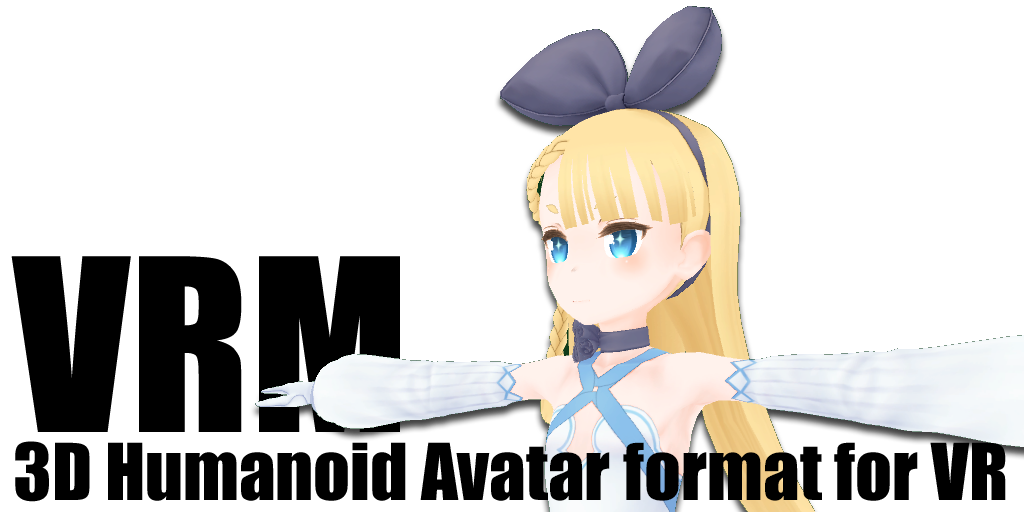# How to use VRM Humanoid in Babylon.js## What is VRM?

“VRM” is a file format for handling 3D humanoid avatar (3D model) data for VR applications. It is based on glTF2.0. Anyone is free to use it.

In addition, a standard implementation (UniVRM) in c# that can import and export VRM file in Unity is released as open source.

Additional informations can view at vrm.dev.

## How to load VRM file in BabylonJS?

You can load VRM file in BabylonJS that uses babylon-vrm-loader, which is community-made BabylonJS glTF Loader Extension for `VRM`.

It supports `.vrm` and `.vci` file loading.

## What can do?

For example, once write some animation code, all VRM models can animate it!``````// example code
vrmManager.humanoidBone.leftShoulder.rotationQuaternion = Quaternion.FromEulerAngles(
Math.sin(Math.PI / 4 * (elapsedTime / 200)),
0,
Math.PI / 3.5,
);
vrmManager.humanoidBone.rightShoulder.rotationQuaternion = Quaternion.FromEulerAngles(
Math.sin(Math.PI + (Math.PI / 4 * (elapsedTime / 200))),
0,
-Math.PI / 3.5,
);
vrmManager.humanoidBone.leftUpperLeg.rotationQuaternion = Quaternion.FromEulerAngles(
Math.sin(Math.PI / 4 * (elapsedTime / 200)),
0,
0,
);
vrmManager.humanoidBone.rightUpperLeg.rotationQuaternion = Quaternion.FromEulerAngles(
Math.sin(Math.PI + (Math.PI / 4 * (elapsedTime / 200))),
0,
0,
);
vrmManager.humanoidBone.leftLowerLeg.rotationQuaternion = Quaternion.FromEulerAngles(
-Math.PI / 6,
0,
0,
);
vrmManager.humanoidBone.rightLowerLeg.rotationQuaternion = Quaternion.FromEulerAngles(
-Math.PI / 6,
0,
0,
);
``````

BabylonJS standard animation mechanism also can be used.

For instance, you can make pose to any VRM.

## How to use?

On browser example is here.

On BabylonJS Playground example is https://playground.babylonjs.com/#K5W35Y -

On npm/yarn with webpack...

``````\$ npm install --save @babylonjs/core @babylonjs/loaders babylon-vrm-loader
# or
``````
``````import * as BABYLON from '@babylonjs/core'

// has side-effect
// ref. https://webpack.js.org/guides/tree-shaking#mark-the-file-as-side-effect-free

// vrmFile is File object retrieved by <input type="file">.

// Update secondary animation
vrmManager.update(scene.getEngine().getDeltaTime());
});

// Model Transformation
vrmManager.rootMesh.translate(new BABYLON.Vector3(1, 0, 0), 1);

// Work with HumanoidBone